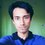# Proof Problem Of The Day - Digits and Sums!

For a positive integer $N$, let $S(N)$ be the sum of digits in the decimal representation of $N$. Let $F(N)$ be the sum of all the numbers obtained by erasing one or more numbers from the right end of $N$.

Prove that $S(N)+9F(N)=N$

Click here to see all the problems posted so far. Keep checking everyday!Note by Mursalin Habib
6 years, 11 months ago

This discussion board is a place to discuss our Daily Challenges and the math and science related to those challenges. Explanations are more than just a solution — they should explain the steps and thinking strategies that you used to obtain the solution. Comments should further the discussion of math and science.

When posting on Brilliant:

• Use the emojis to react to an explanation, whether you're congratulating a job well done , or just really confused .
• Ask specific questions about the challenge or the steps in somebody's explanation. Well-posed questions can add a lot to the discussion, but posting "I don't understand!" doesn't help anyone.
• Try to contribute something new to the discussion, whether it is an extension, generalization or other idea related to the challenge.

MarkdownAppears as
*italics* or _italics_ italics
**bold** or __bold__ bold
- bulleted- list
• bulleted
• list
1. numbered2. list
1. numbered
2. list
Note: you must add a full line of space before and after lists for them to show up correctly
paragraph 1paragraph 2

paragraph 1

paragraph 2

[example link](https://brilliant.org)example link
> This is a quote
This is a quote
    # I indented these lines
# 4 spaces, and now they show
# up as a code block.

print "hello world"
# I indented these lines
# 4 spaces, and now they show
# up as a code block.

print "hello world"
MathAppears as
Remember to wrap math in $$ ... $$ or $ ... $ to ensure proper formatting.
2 \times 3 $2 \times 3$
2^{34} $2^{34}$
a_{i-1} $a_{i-1}$
\frac{2}{3} $\frac{2}{3}$
\sqrt{2} $\sqrt{2}$
\sum_{i=1}^3 $\sum_{i=1}^3$
\sin \theta $\sin \theta$
\boxed{123} $\boxed{123}$

Sort by:

At first let $N=\displaystyle\sum_{k=0}^n a_k 10^k$. We have $S(N)=\displaystyle \sum_{k=0}^n a_k$. Now we calculate and simplify $F(N)$. A little observation is required to find the compact form of $F(N)$

$F(N) = \sum_{i=1}^n \sum_{j=i}^n a_j 10^{j-i}=\sum_{i=1}^{n} \sum_{j=0}^{i-1} a_{i} 10^j=\sum_{i=1}^n\left(a_{i}\sum_{j=0}^{i-1} 10^j\right)=\sum_{i=1}^n a_{i}\dfrac{10^i-1}{9}.$

The rest is straight. Using the last form of $F(N)$ we have

$9F(N)=\sum_{i=1}^n a_i\left(10^i -1\right)=\sum_{i=1}^n \left(a_i10^i-a_i\right)=\sum_{i=1}^n a_i10^i -\sum_{i=1}^n a_i =N-S(N)$

and the result follows. $\square$

NOTE: If you're wondering how I reformed the sum of $F(N)$ in the third step above, say $N=3265$. Then clearly

$F(3625)=326+32+3.$

This is the form of the first sum. Now break further into

\begin{aligned} F(3625) &=&326+32+3 \\ &=&(300+20+6)+(30+2)+3 \\ &=&(300+30+3)+(20+2)+6 \\ &=&3(10^2+10^1+10^0)+2(10^1+10^0)+6\cdot 10^0. \end{aligned}

Observe the number of occurrences of the powers of $10$ for the $n$th digit and generalize to get the second sum.

- 6 years, 11 months ago

Great job!

Another way of doing it is inducting on the number of digits of $N$.

Clearly the statement is true for any one digit-number.

Assume that the statement is true for any $k$-digit number.

Now for any $(k+1)$- digit number $b$, let $b=10a+c$ where $a$ is a $k$-digit number and $c$ is a one-digit number.

Notice that, $S(b)=S(a)+c$ and $F(b)=F(a)+a$

So, $S(b)+9F(b)=S(a)+c+9F(a)+9a$.

From the induction hypothesis, $S(a)+9F(a)=a$. Substituting that, we get,

$S(b)+9F(b)=10a+c=b$.

So the result holds for all positive integers $N$.

- 6 years, 11 months ago

BTW, someone's downvoting every damn comment he's passing by. -_-

- 6 years, 11 months ago

I don't mind being downvoted.

- 6 years, 11 months ago

Neither do I. But I do mind being spammed.

- 6 years, 11 months ago

At first it seems rather tedious. However, if we prove by induction on the number of digits the calculations will magically disappear!

When number of digits is 1, $F(N)=0, S(N)=n$, done.

Suppose it was true for all $k$ digit numbers. Consider a $(k+1)$ digit number $X$ with last digit $m$. Let $Y$ be the $k$ digit number without $m$. Then $X=10Y+m$. Hence $S(X)+9F(X)=S(Y)+m+9F(Y)+9Y$ (since we missed out $Y$ when calcualting $F(Y)$).

But by inductive hypothesis, $S(Y)+9F(Y)=Y$, hence $S(X)+9F(X)=Y+m+9Y=10Y+m=X$ and we are done.

- 6 years, 11 months ago

Sorry, I did not see Mursalin's comment below. I just realized it was a repetition.

- 6 years, 11 months ago

Interesting.

- 6 years, 10 months ago